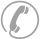### 17333258710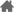您现在的位置：安徽成人高考 > 成考高起专 > 数学（理） > 模拟试题 >

# 2023年安徽成人高考高起专《数学理》备考模拟题一1.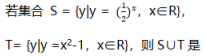A Ø

B T

C S

D 有限集

2.实数m，n满足m2+n2≠0的充分必要条件是

A m≠0且n≠0

B m≠0或n≠0

C m≠0

D n≠0

3.若α+β=π/4，则（1+tanα)(1+tanβ)=

A 1

B -1

C 2

D -2

4.下列函数中，定义域为全体实数的是

A y = lgx2

B y =1/x

C y =1/sinx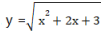5.函数y=3x =与y =-3-x的图像关系是

A 关于x轴对称

B 关于原点对称

D 同一条曲线

6.在等比数列{an}中，a3 • a4 = 5，则a1• a2 • a5 • a6=

A 25

B 10

C -25

D -10

7.从1，2,3,4,5中，每次取出两个不同的数字组成两位数，其中奇数的个数是

A 6

B 8

C 10

D 12

8..已知sinα=-3/5,且a∈(3π/2,2π)，则tan2α的值等于

B 21/8

C -3/2

9.偶函数f(x）在x∈[2,4]上单调递减，则a =f(log1/28)与b =f(-π)的关系是

A a>b

B a<b

C a = b

D 以上都不

10.设log34•log48•log8m=log416，则m =

A 9/2

B 9

C 18

D 27

11.平行于直线x-y-2=0,并且与它的距离为2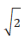的直线方程是

A x-y+2 = 0

B x-y-6 = 0

C x-y+2=0或x-y-6 = 0

D x-y-2 =0或x-y+6 =0

12.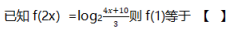A 1/2

B 1

C log214/3

D 2

0人参与，0条评论

### 全部评论(0)## 高起专教材推荐

•微信扫码添加老师一对一咨询解答

•安徽成考微信公众号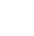学历提升报名热线：17333258710